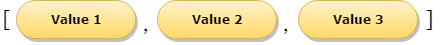## Swift Tutorial Index

Till now, we have seen and used the basic data types. Though these types are handy for declaring variables, constants, or a return type for a function; they are restrained by the fact that these types can store only one value at a given time. So for dealing with multiple values under a single name programmer have to use the Arrays. In this chapter, you will learn about how to work with arrays and deal with them properly using specific syntax.

## What are The Arrays in Swift?

Swift arrays are applied for storing multiple values in ordered lists of any specific type. Swift set up strict checking mechanism which does not allow programmers to enter or insert any wrong type in an array even by mistake, i.e., arrays in swift programming are specific about the kinds of values they can store. The same value can appear in an array multiple times at different positions. The swift arrays vary from Objective-C's NSArray and NSMutableArray classes, which can store any object and do not provide any information about the nature of the objects they return. In Swift, the type of values that a particular array can store is always made clear, either through an explicit type annotation, or through type inference, and does not have to be a class type. Swift arrays are type safe and are always clear about what they may contain.

## Shorthand syntax for Arrays

The swift array can also be written in this format as - Array<SomeType>, where 'SomeType' is the type that the array is going to store. Programmers can also write the type of an array in shorthand form as SomeType[]. Although the two mentioned forms are functionally identical, the shorthand form is preferred more than the other one. If programmers assign an already existing array to a variable, then its always mutable. This means the programmer can change it by adding, removing, or altering its items. But in case if the arrays are assigned to a constant, then that array is immutable, and its size and contents cannot be changed.

Example:

``````import Cocoa

var arr = [Int](count: 4, repeatedValue: 6)

var arrstart = arr

println( "Starting value of array is \(arrstart)" )

println( "Second element of array is \(arr)" )

println( "Third element of array is \(arr)" )

println( "Fourth element of array is \(arr)" )``````

Output:

```Starting value of array is 6
Second element of array is 6
Third element of array is 6
Fourth element of array is 6```

## Array Literals

You can initialize an array with an array literal, which is a shorthand way to write one or more values as an array collection. An array literal is written as a list of values, separated by commas, surrounded by a pair of square brackets. Here is how they look like:## Accessing and Modifying Arrays

Arrays can be easily modified using the append() method or by using the addition assignment operator (+=) for inserting new items at the end of an array.

Example:

``````var arr = [Int]()

arr.append(100)

arr.append(200)

arr += 

var arr2 = arr

print( "First element of array is \(arr2)" )

print( "Second element of array is \(arr)" )

print( "Third element of array is \(arr)" )``````

Output:

```First element of array is 100
Second element of array is 200
Third element of array is 300```

## Iterating Over the Arrays:

Programmers can iterate over the entire set of values in an array with the for-in loop. Here is an example to show how this concept works.

``````import Cocoa

var arr = [String]()

arr.append("Milk")

arr.append("Eggs")

arr.append("Flour")

arr.append("Baking Powder")

arr.append("Fruits")

arr += ["Breakfast"]

for item in arr {

println(item)

}``````

When programmers need the integer index of each item as well as its value, programmers need to use the global enumerate function to iterate over the array instead. These enumerate function returns a tuple for each item in the array composed of the index and the value for that item. You can decompose the tuple into temporary constants or variables as part of the iteration. Here how it goes:

``````for (index, value) in enumerate(shoppingList) {

println("Item \(index + 1): \(value)")

}``````

## The empty Property

Programmers usually use the read-only empty property of arrays for finding out whether an array is empty or not. Here is a simple example showing the use of the empty property.

``````import Cocoa

var arr1 = [Int](count:2, repeatedValue: 2)

var arr2 = [Int](count:3, repeatedValue: 4)

var arr3 = [Int]()

var arr4 = [Int](count:3, repeatedValue: 6)

println("arr1.isEmpty = \(arr1.isEmpty)")

println("arr2.isEmpty = \(arr2.isEmpty)")

println("arr3.isEmpty = \(arr3.isEmpty)")

println("arr4.isEmpty = \(arr4.isEmpty)")``````

Output:

```arr1.isEmpty = false
arr2.isEmpty = false
arr3.isEmpty = true
arr4.isEmpty = false```